1.1 按照运算的类型来分：

1.2 按照支持的函数来分：

### 2.同态加密步骤

2.1 非对称加密步骤

2.2 几个关于同态加密Paillier 算法的问题：

a) 如何生成密钥？

b) 如何加密？

2.3 其他资源

### 2.细节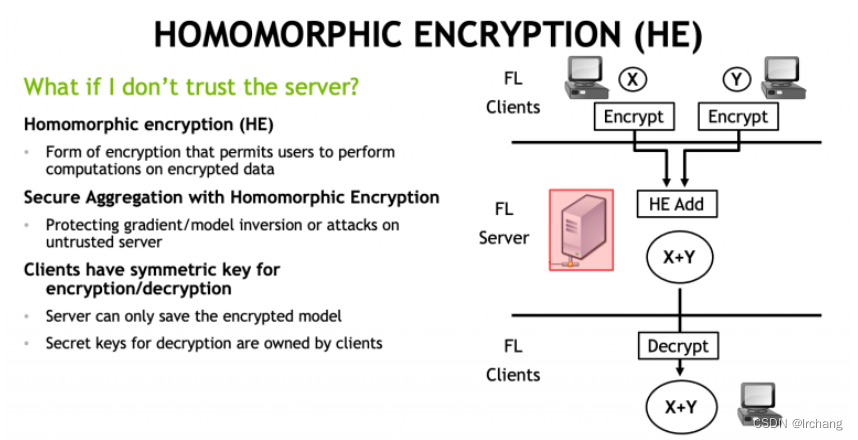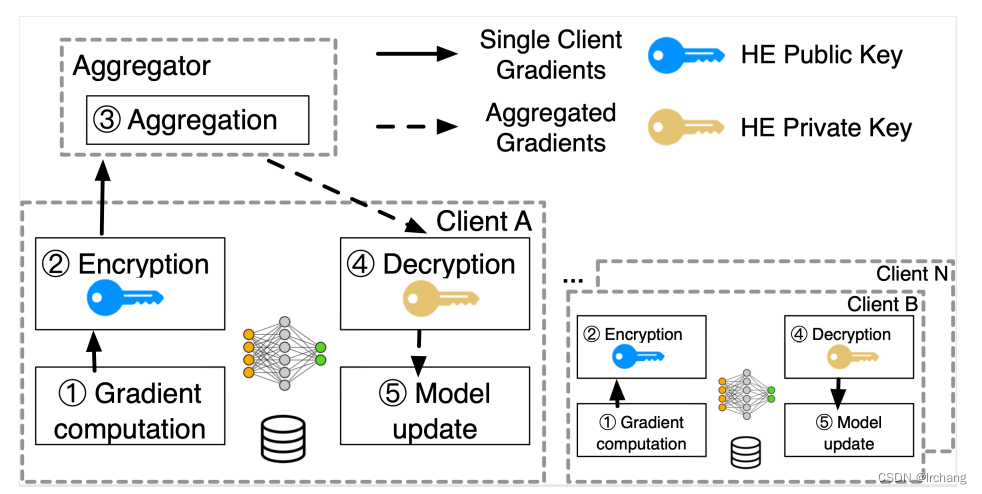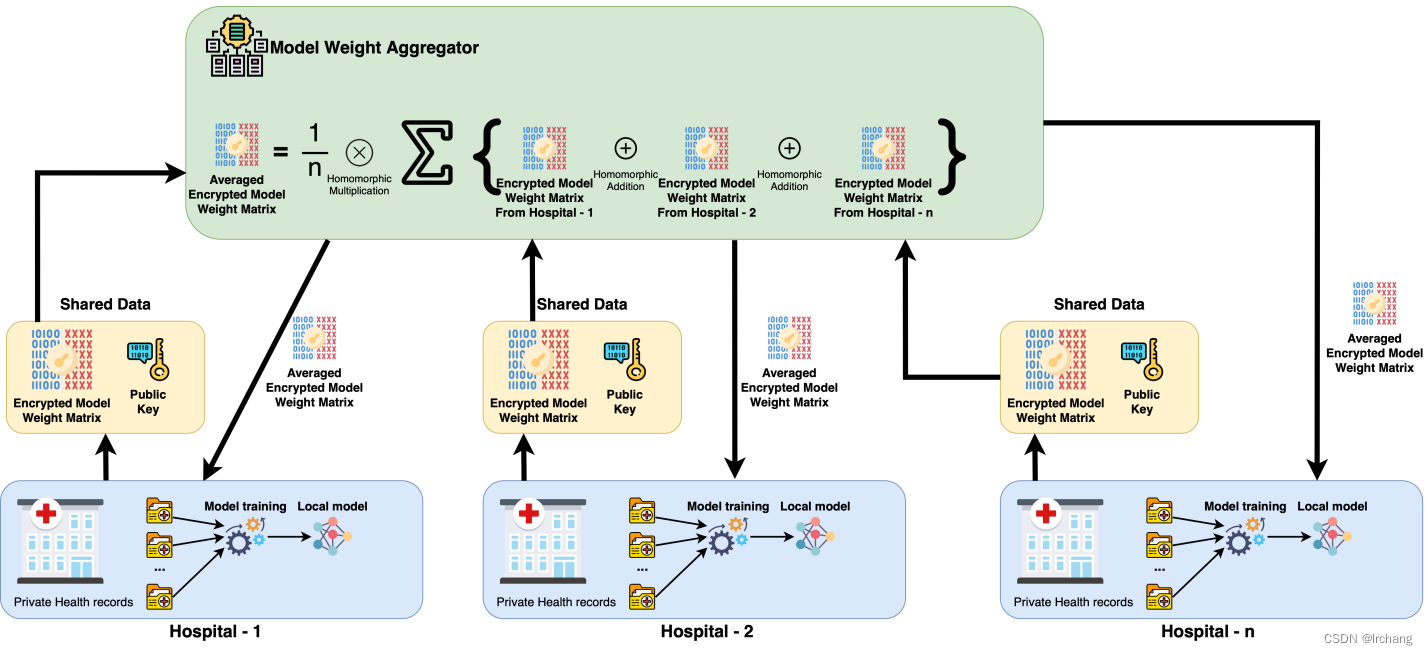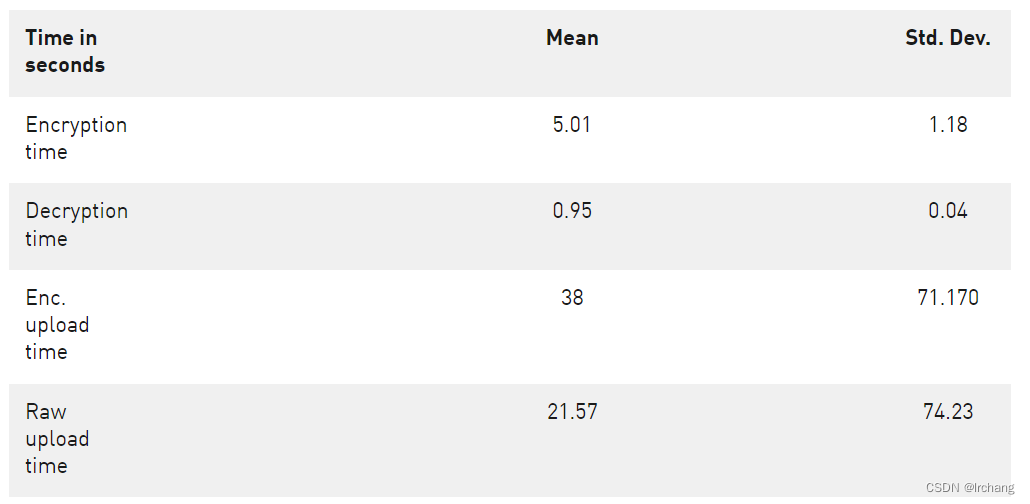Wibawa, Febrianti, et al. “Homomorphic Encryption and Federated Learning based Privacy-Preserving CNN Training: COVID-19 Detection Use-Case.” arXiv preprint arXiv:2204.07752 (2022).

### 1.手写代码

import random

'''
Euclid's algorithm for determining the greatest common divisor
Use iteration to make it faster for larger integers
'''

def gcd(a, b):
while b != 0:
a, b = b, a % b
return a

'''
Euclid's extended algorithm for finding the multiplicative inverse of two numbers
'''

def multiplicative_inverse(e,r):
for i in range(r):
if((e*i)%r == 1):
return i

def is_prime(num):
if num == 2:
return True
if num < 2 or num % 2 == 0:
return False
for n in range(3, int(num ** 0.5) + 2, 2):
if num % n == 0:
return False
return True

def generate_keypair(p, q):
if not (is_prime(p) and is_prime(q)):
raise ValueError('Both numbers must be prime.')
elif p == q:
raise ValueError('p and q cannot be equal')
# n = pq
n = p * q

# Phi is the totient of n
phi = (p - 1) * (q - 1)

# Choose an integer e such that e and phi(n) are coprime
e = random.randrange(1, phi)

# Use Euclid's Algorithm to verify that e and phi(n) are comprime
g = gcd(e, phi)
while g != 1:
e = random.randrange(1, phi)
g = gcd(e, phi)

# Use Extended Euclid's Algorithm to generate the private key
d = multiplicative_inverse(e, phi)

# Return public and private keypair
# Public key is (e, n) and private key is (d, n)
return ((e, n), (d, n))

def encrypt(pk, plaintext):
# Unpack the key into it's components
key, n = pk
# Convert each letter in the plaintext to numbers based on the character using a^b mod m
cipher = [(ord(char) ** key) % n for char in plaintext]
# Return the array of bytes
return cipher

def decrypt(pk1, pk2, ciphertext):
# Unpack the key into its components
key, n = pk1, pk2
# Generate the plaintext based on the ciphertext and key using a^b mod m
plain = [chr((char ** key) % n) for char in ciphertext]
# Return the array of bytes as a string
return ''.join(plain)

if __name__ == '__main__':

p = int(input("输入一个素数: "))
q = int(input("再输入一个素数: "))

public, private = generate_keypair(p, q)
print("为你生成的公钥是：", public)
print("为你生成的私钥是：", private)

message = input("输入需要加密的数据: ")
encrypted_msg = encrypt(public, message)
print("您获得的密文是：", ''.join(map(lambda x: str(x), encrypted_msg)))

privatee = []
privatee.append(int(input('输入您的私钥前排序列')))
privatee.append(int(input('输入您的私钥后排序列')))

print('密文的解密结果为：', decrypt(privatee, privatee, encrypted_msg))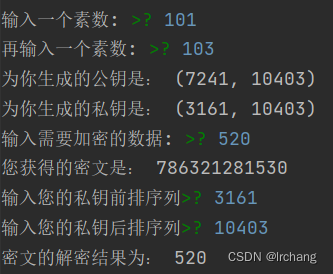### 2.调包实现乘法同态加密

import rsa
# 1.生成密钥
(public_key, private_key) = rsa.newkeys(512)
print('公钥为：{}  私钥为：{}。'.format(public_key, private_key))

# 待传输的信息，编码方式为utf-8
emessage = 'Hello world!'.encode('utf-8')

# 3.使用公钥加密信息
crypto = rsa.encrypt(emessage, public_key)
print(crypto)

# 4.使用私钥解密信息
dmessage = rsa.decrypt(crypto, private_key)
print(dmessage.decode('utf8'))


公钥为：PublicKey(8095059374219196630454198555684841855555779377170836284535186351217329395948699458601330730050809264266228630028651115426719314399559600212173369081099979, 65537)  私钥为：PrivateKey(8095059374219196630454198555684841855555779377170836284535186351217329395948699458601330730050809264266228630028651115426719314399559600212173369081099979, 65537, 3373672226193584045159154754587791409444970049417383332156027050533270024472236092302389157206342283683740184679587325263536984653117242945602120034664897, 7238673580383412616612813154973643099381838015408521462886068127059748767382973867, 1118307005327131164182883905657023822538740327140066922538214293760080737)。
b'A\x10_\x8cUC\xe2\xaf\xda\x18\x9d\xc7nr1}\xcd3\xdfQ\xa6\x9f\xb2W<\x82\xdcN\xa3\xc6-\x9f\xa0i\x03\xd1\xa8[\x98\xd7\xcelm\xea\xa8\xb15S\xd3\x19\xe1\xd4\x8d0\x956\x85rE\xce\n\xb2H\xf5'
Hello world!



2022年5月30日前更新

### 推荐阅读